﻿ Optimal Control for the Voltage Regulation of a Power Line Including an Interconnection with a Wind Park and FACTS

### Optimal Control for the Voltage Regulation of a Power Line Including an Interconnection with a Wind ...

N. Schinas, A. Moullas

## Optimal Control for the Voltage Regulation of a Power Line Including an Interconnection with a Wind Park and FACTS

N. Schinas1,, A. Moullas1

1Department of Electrical Engineering, Technological Educational Institute of Western Greece, Patras, Greece

### Abstract

In this paper the voltage regulation of a grid bus is studied. The bus accepts real power from a wind park through a medium voltage cable. The changes of the wind speed result in large alterations in the exchange of real and reactive power between the cable and the grid. As a result, the voltage of the bus is not stable. The installation of a Static Var Compensator(SVC) is a rational choice for the stabilization of the voltage. The SVC needs a controller which according to the difference of the measured voltage from its nominal value, it defines the equivalent susceptance of the SVC. So, the right amount of reactive power between the grid and the SVC is achieved. Two design techniques for the controller are proposed, both based on the theory of optimal control. The full theory of the optimal control used in the design procedure, is given clearly. In the first place, the nonlinear model is linearized in two ways and then the mathematical principles of optimal control are applied. The second input of the linearized model, regarded as disturbance, has been taken into account in the design procedure. The proposed application of the optimal control theory has resulted in controllers that can be regarded approximately as PI controllers andso, their implementation is very easy. The whole nonlinear system has been simulated in SIMULINK so as the response of the proposed system to be judged. The results are satisfactory. Despite the large variations in the exchange of real and reactive power between the wind park and the grid, the variation of the grid voltage is under 1.5%.

• N. Schinas, A. Moullas. Optimal Control for the Voltage Regulation of a Power Line Including an Interconnection with a Wind Park and FACTS. American Journal of Electrical and Electronic Engineering. Vol. 4, No. 1, 2016, pp 9-15. http://pubs.sciepub.com/ajeee/4/1/2
• Schinas, N., and A. Moullas. "Optimal Control for the Voltage Regulation of a Power Line Including an Interconnection with a Wind Park and FACTS." American Journal of Electrical and Electronic Engineering 4.1 (2016): 9-15.
• Schinas, N. , & Moullas, A. (2016). Optimal Control for the Voltage Regulation of a Power Line Including an Interconnection with a Wind Park and FACTS. American Journal of Electrical and Electronic Engineering, 4(1), 9-15.
• Schinas, N., and A. Moullas. "Optimal Control for the Voltage Regulation of a Power Line Including an Interconnection with a Wind Park and FACTS." American Journal of Electrical and Electronic Engineering 4, no. 1 (2016): 9-15.

 Import into BibTeX Import into EndNote Import into RefMan Import into RefWorks

123
Prev Next

### 1. Introduction

Many scientific papers regarding voltage control in power systems can be found in the literature. For instance, there are many new control schemes that deal with voltage control on large - scale power systems () or distribution power systems ([2, 3]). The integration of renewable energy systems into the main grid makes the voltage even more unstable according to the fluctuations of the produced energy and the load. One solution to the stabilization of the voltage is the installation of FACTS devices in power systems with wind generation ([4, 5, 6]). Many schemes are presented in these papers for the control of various FACTS devices and the right performance of the grid voltage, although it may be difficult for some of them to be implemented due to their complex form.

In this paper, a specific interconnection between a wind power station and the main grid is studied and simple PI controllers for the stabilization of the voltage are designed, according to the optimal control theory.

Many applications of wind parks require off - shore installations that means interconnection with the main grid through submarine cables. As a result, the grid voltage is susceptible to alterations from the rated value because of the significant changes of the real and reactive power being interchanged between the grid and the cable.

In this paper a Static Var Compensator (SVC) scheme equipped with a specific controller is proposed which is able to maintain the grid voltage constant despite the large alterations of the wind speed. The design of the controller is based on optimal control theory and finally a simple PI controller can be applied.

The system under study is shown in Fig. 1. It can be seen the bus of the grid to which the cable of the wind park is connected. The SVC is connected to the same bus. The goal of the controller of the SVC is to keep the voltage of the bus as constant as possible under large variations of real and reactive power from the cable into the grid. The rated voltage of the cable is 49 kV so it is connected to the main grid through a transformer 150/49 kV. The wind park (WP) consists of 26 doubly fed wind turbines (WT) using asynchronous generators. The rated power of each WT is 1.5 MVA and the rated voltage 575 V. For simplicity reasons there is a central transformer 49 kV/ 0.575 kV for the interconnection of the WP to the cable. In the Appendix, there are the values of all the parameters of the system. The whole nonlinear system has simulated in the SIMULINK software using its libraries for the main components such as the WT, the cable, the SVC etc.Download asVeiw figureFigures index
Figure 1. System under study

### 2. Description and Modeling of the System

In this paragraph, we develop the original nonlinear mathematical equations of the various components of the system and then we linearize them around the operating point. The operating point has been chosen to be the steady state of the system when the WP produces its maximum real power S0 = 40 MW under rated voltages. For the whole system it has been decided to be Sb = 100 MVA. So in p.u. it is: S0 = 0.4. The voltage of the bus under study is Vand the voltage of the bus at the output of the wind park is VWT. For the operating point it is V0 = V0WT = 1 in p.u.

For short lines of length 100 km or less, the total shunt admittance is very small and can be neglected. So the equivalent electric circuit of the line is the circuit given in Figure 2a. The relationship between sending-end voltage (Vs) and receiving-end voltage (Vr) can be written as: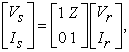where Is, Ir are the sending-end and receiving-end currents respectively.

For, lines more than 100 km long, or cables, charging currents due to the shunt capacitance can not be neglected. The capacitance is uniformly distributed throughout the length of the cable, but for cables not too long, the nominal – πrepresentation can be used. In this method, the total line capacitance Y is divided into two equal parts which are lumped at the sending and receiving ends of the cable. The equivalent circuit is given in Figure 2b. From Figure 2b, it is: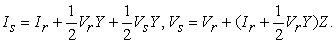Finally, we have in matrix form: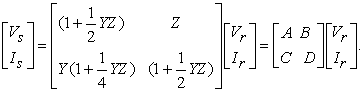In this form, the constants A,B,C,D of the cable have been included.Download asVeiw figureFigures index
Figure 2a. Equivalent circuit of a short lineDownload asVeiw figureFigures index
Figure 2b. Cable representation

The cable carries a current Ica (= Ir) towards its connection to the grid bus and accepts a current IWT (= Is) from the wind park. From the values of the Table A2 in the Appendix, the model of the cable in the s-plane it is: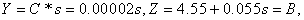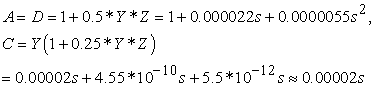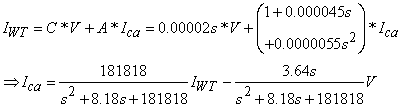For Vb = 49 kV it is Ib = 1178 A. So the previous equation in pu is written in linearized form as: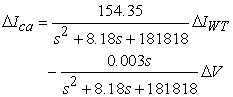(1)

The grid is simulated through a voltage source E and an inductor equal to Xgrid = 0.18 H. This value corresponds to a short-circuit capacity of 400 MVA which is ten times the rated power of the wind park. We suppose a ‘weak’ node of the system because in this occasion, the voltage is more sensitive to the alterations of Ica. The internal voltage E is not a parameter in the linearized model, and if we notice that Ib = 385 A in 150 kV it finally is in pu: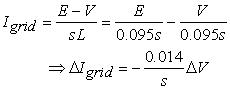(2)

The bus voltage regulation at a bus conventionally is achieved through static shunt reactive power compensationwhich means the installation of a combination of capacitors at the bus. Modern SVC schemes use power electronics technology and so fast and smooth adjustment of the voltage is achieved. They may use either thyristor controlled reactors (TCRs) or thyristor switch capacitors (TSCs). In any case, the SVC can be viewed as a reactive power source from the grid side. With the right pulsation procedure, the equivalent susceptance BSVC of the SVC is adjusted in order the right amount of reactive power to be interchanged between the SVC and the grid for the successful regulation of the bus voltage. So, it isgenerally: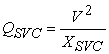and in steady state operation it will be in p.u.: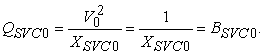The steady state power flow model of the cable is: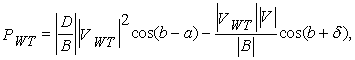where δ is the angle between the voltages VWT and V, PWT is the real power of the WP and the parameters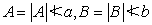are the A,B,C,D parameters from the equivalent Πcircuit of the cable. From the rated parameters of the cable it is: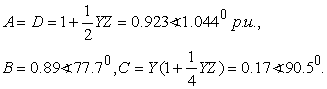For maximum real power production from the WP (P = 40 MW or 0.4 p.u.) and rated voltages (all equal to 1 p.u.) we have from the above equation that δ0 = 20.50. The steady state reactive power Qca0 from the cable into the grid is given by: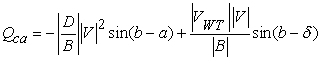and by substitution Qca0 = -0.066 p.u., which means that the cable absorbs reactive power from the grid.

The real power coming from the WP inserts into the grid, so from the equations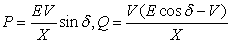we can compute that the reactive power from the grid is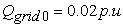. So, in steady state operation, the equation of the reactive power flows at the bus gives: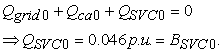The current from the SVC generally is equal to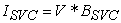, (), so the linearization gives: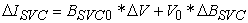. In order a simple delay in the pulsation of the SVC to be taken into consideration, the previous equation will become: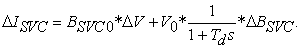The time delay Td is taken equal to 3 ms, so the linearized form of the alteration of the SVC current, finally becomes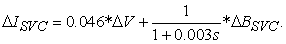(3)

Applying Kirchhoff’s law regarding the bus currents we have: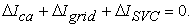Substituting from (1), (2), (3) and after some algebra, we finally result in the following basic equation that gives the linearized model of the bus voltage: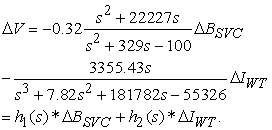(4)

This equation describes the dynamic behavior of the bus voltage (output in our model) according to the susceptance of the SVC (input) and the current from the WP (disturbance). Our control system will be designed according to this model. Besides, the reactive power from the SVC will be: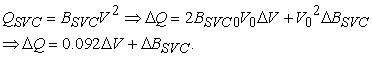(5)

### 3. Optimal Control Design

3.1. SimpleNormalized Model of the Systemand Controller Design

In order a fast response to be achieved, the previous linear model of the system is adjusted so that it contains the normalized values of the corresponding parameters. The normalized value of ΔV is assigned as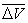and is equal to: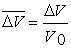. Similarly the normalized values of the rest of the parameters have been defined as: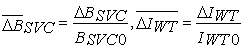. From (4) and neglecting the third order of s we have: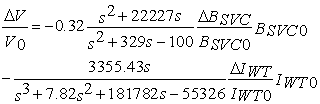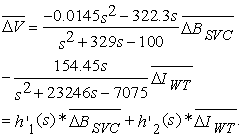(6)

The normalized equation of the SVC reactive power is derived from (5) as: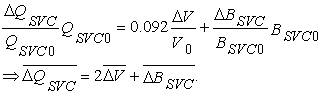(7)

The first simple design procedure is based on the above model and only on the input-output transfer function h’1 (s), so the disturbance parameter has not been taken into consideration.

In state space equations, the transfer function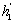is transformed into the matrices: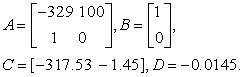(8)

The main element used in the optimization criterion is the reactive power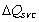from the SVC and so the optimization criterion is defined as: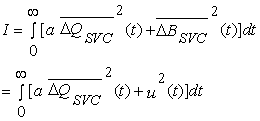since the input u to the control system is the susceptance of the SVC. From (6) and (7) it is: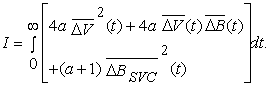(9)

From the (8) it actually is: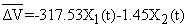,where x1(t), x2(t) are the state variables of the system. By substitution into (9), we have: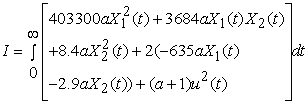which is of the form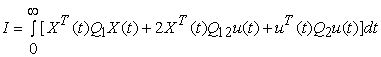([8, 9]). Apparently, the weighting matrices are: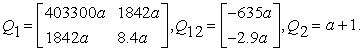The problem is solved through solving the relevant Riccati equation: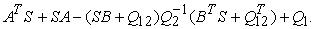The state feedback L is then: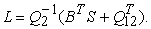The L has been calculated through MATLAB software for various values of the parameter a. The control law is u = -Lx. Then an equivalent series controller has been calculated in order the final control system is of the form depicted in Fig.3. For instance, for a=1, the equivalent series controller which was derived from the optimal state feedback law, is: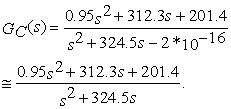Download asVeiw figureFigures index
Figure 3. Simple control loop for the design of the SVC controller

We can use this controller, but if we neglect the second order of s, then we conclude to a simple PI controller with parameters Kp = 0.96 and KI = 0.62 which is very easy to be implemented in a microprocessor. So, we see that through the optimal theory we reached in a simple PI controller for our application. Other values for ‘a’ give not very different values for the parameters of the PI controller, for example, if a=1000 then Kp = 1.6 and KI = 0.62. As we will see in the next paragraph, the simulation of the nonlinear system showed that there are no significant differences in the response of the system for various values of the parameter ‘a’.

3.2. Augmented Normalized Model and controller Design

In the previous design technique, the controller decides about the proper susceptance of the SVC having taken into consideration only the difference between the rated voltage and the actual one (transfer function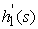).

In order the dynamics of the presence of the disturbance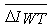to be taken into consideration, an augmented system is proposed for the design of the optimal controller. The state space model of the system given by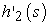is: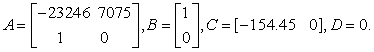The augmented system in state space will be: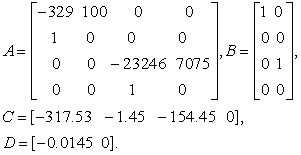(10)

The state-variables matrix x(t) contains the four state variables of the system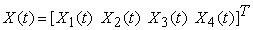and the vector u for the input of the system is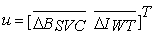.The optimization criterion now is the same as before, but the input now will be named as u1 as only the parameter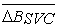is the control signal. So: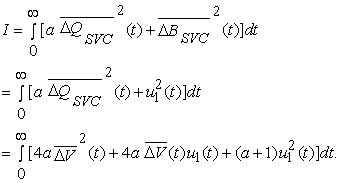From (10) it is:By substitution and after some algebra, we finally result in: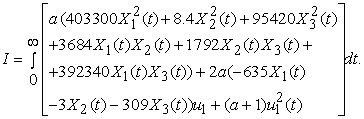The weighting matrices now are: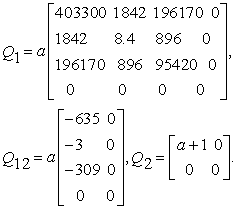The problem is solved through solving the relevant Riccati equation for the calculation of the state feedback gain L. The L has been calculated through MATLAB software for various values of the parameter ‘a’. As before, an equivalent series controller has been calculated in order the final control system is of the form depicted in Fig.3. For instance, for a=1, the equivalent series controller which was derived from the optimal state feedback law, if we neglect high orders of s, is: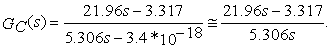Apparently, this can be implemented again easily as a PI controller with Kp = -4.14 and KI =0.62. One can notice that, the design procedures result in controllers of the same form and the KI parameter is the same in both of the design schemes with normalized values for the model parameters and for the same value of ‘a’.

### 4. Nonlinear System Simulation Results

The system under study as is depicted in Fig. 1 has been simulated in the SIMULINK software. The nonlinear models for the DFIM and SVC have been taken from the software library. The rated values for the machines are listed in the Appendix.

In the first case, we test the response of the system under severe changes of the wind speed which bring about huge alterations of real and reactive power from the cable into the grid. As a result, the voltage of the grid changes. The proposed optimal controllers of the SVC take on to adjust the grid voltage to its nominal value. In Fig. 4 the wind speed is depicted. The wind speed increases rapidly from 8 m/s to 14 m/s at t = 80 sec and then decreases to 8 m/s at t = 150 sec.Download asVeiw figureFigures index
Figure 4. Wind speed waveform

In Figure 5 the real power coming from the wind park is shown which alters according to the wind speed(p.u. refers to the rated power of the wind park).Download asVeiw figureFigures index
Figure 5. Real Power of the Wind Park

In Figure 6 (a) we see the response of the grid voltage with the optimal controller designed according to the simple normalized model for a =100, while in Figure 6 (b) the response with the optimal controller according to the augmented normalized model.Download asVeiw figureFigures index
Figure 6. Grid voltage response under the two optimal controllersDownload asVeiw figureFigures index
Figure 7. Reactive power of SVC under the two optimal controllers

In Figure 7 (a) we see the change in the reactive power of the SVC due to the first controller and in Figure 7 (b) due to the second controller.

Generally, the response of the system is satisfactory in both situations and with both of the control schemes.Download asVeiw figureFigures index
Figure 8. Grid voltage response with the simple model for a = 1000

We can see that the simple normalized model results in a controller that has small overshoots but with slow response. Under a severe wind speed change, the variation of the bus voltage is hardly 1.5%. On the contrary, the augmented normalized method results in a controller with larger overshoots but much faster than the first one (about half the time of the previous controller). The final choice depends on the demands.

Finally, in Figure 8 there is the response of the grid voltage with the simple normalized model for a = 1000.

We can see that there is no significant change in comparison with the Figure 6a. The only subtle difference is that the peaks of the transient periods are smaller in this case.

### 5. Conclusions

A high voltage grid bus is considered in this paper to which a wind park is connected through a medium voltage cable. The severe changes of the real and reactive power coming from the cable into the grid make the voltage unstable. The installation of an SVC improves the voltage response. The SVC is controlled through two different proposed controllers which have been designed according to the theory of optimal control. The theory results in simple PI controllers which can be implemented easily. The response of the system is satisfactory and the voltage variation due to the changes of the power from the wind park can be confined between +/- 1.5% in a time-limited transient period.

### References

  Vu, H., Pruvot, P., Launay, C., and Harmand, Y. (2002), An improved voltage control on large – scale power system, IEEE Transactions on Power Systems, 11(3), pp. 1295-1303.In article View Article  Senjyu, T., Miyazato, Y., Yona, A., and Urasaki, N. (2008), Optimal distribution voltage control and coordination with distribution generation, IEEE Transactions on Power Delivery, 23(2), pp:1236-1242.In article View Article  Novalio, D., Biswarup, D., and Jaydev, S. (2015), Robust voltage regulation in unbalanced radial distribution system under uncertainty of distribution generation and loads, International Journal of electrical Power & Energy Systems, 73, pp:516-527.In article View Article  Dawood, A. (2016), DSTATCOM modelling for voltage stability with fuzzy logic PI controller, International Journal of electrical Power & Energy Systems, 76, pp: 129-135.In article View Article  Elmitwally, A., and Eladl, A. (2016), Planning of multi-type FACTS devices in restructured power systems with wind generation, International Journal of electrical Power & Energy Systems, 77, pp:33-42.In article View Article  Khezri, R., and Bevrani, H. (2015), Voltage performance enhancement of DGIG-based wind farms integrated in large-scale power systems: Coordinated AVR and PSS, International Journal of electrical Power & Energy Systems, 73, pp:400-410.In article View Article  Wang, X.F., Song, Y., and Irving, M.(2008), Modern Power System Analysis, Springer, New York.In article View Article  Astrom, K.J., and Wittenmark, B.(2013),Computer Controlled Systems, Dover, New York.In article  Dorf, R.C., and Bishop, R.H. (2011), Modern Control Systems, Prentice-Hall, London.In article PubMed  Vittal, V., and Ayyanar, R. (2013), Grid Integration and Dynamic Impact of Wind Energy, Springer, New York.In article View Article  Abad, G., Lopez, J., Rodriguez, M.A., Marroyo, L., and Iwanski, G. (2011), Doubly Fed Induction Machine, IEEE Press, New Jersey.In article View Article  Gonen, T. (2014), Electrical Power Transmission System Engineering, Analysis and Design, CRC Press, New York.In article